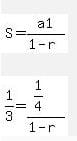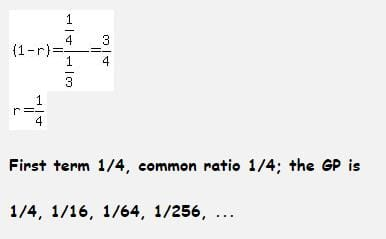Test: Arithmetic And Geometric Progressions - 3

# Test: Arithmetic And Geometric Progressions - 3

Test Description

## 40 Questions MCQ Test Quantitative Aptitude for CA CPT | Test: Arithmetic And Geometric Progressions - 3

Test: Arithmetic And Geometric Progressions - 3 for CA Foundation 2022 is part of Quantitative Aptitude for CA CPT preparation. The Test: Arithmetic And Geometric Progressions - 3 questions and answers have been prepared according to the CA Foundation exam syllabus.The Test: Arithmetic And Geometric Progressions - 3 MCQs are made for CA Foundation 2022 Exam. Find important definitions, questions, notes, meanings, examples, exercises, MCQs and online tests for Test: Arithmetic And Geometric Progressions - 3 below.
Solutions of Test: Arithmetic And Geometric Progressions - 3 questions in English are available as part of our Quantitative Aptitude for CA CPT for CA Foundation & Test: Arithmetic And Geometric Progressions - 3 solutions in Hindi for Quantitative Aptitude for CA CPT course. Download more important topics, notes, lectures and mock test series for CA Foundation Exam by signing up for free. Attempt Test: Arithmetic And Geometric Progressions - 3 | 40 questions in 40 minutes | Mock test for CA Foundation preparation | Free important questions MCQ to study Quantitative Aptitude for CA CPT for CA Foundation Exam | Download free PDF with solutions
 1 Crore+ students have signed up on EduRev. Have you?
Test: Arithmetic And Geometric Progressions - 3 - Question 1

### In an A.P., if common difference is 2, Sum of n terms is 49, 7th term is 13 tthen then n = _________.

Test: Arithmetic And Geometric Progressions - 3 - Question 2

### If 8th term of an A.P. is 15, then sum of its 15 terms is

Test: Arithmetic And Geometric Progressions - 3 - Question 3

### If the sum of n terms of an A.P. be 2n2 + 5n, then its 'nth' term is

Test: Arithmetic And Geometric Progressions - 3 - Question 4

If 'n' arithmetic means are inserted between 7 & 71 and 5th arithmetic mean is 27, then 'n' is equal to:

Test: Arithmetic And Geometric Progressions - 3 - Question 5

If the sum of n terms of an A.P. be 3n2 - n and its common difference is 6, then its first terms is:

Test: Arithmetic And Geometric Progressions - 3 - Question 6

In a G.P. the sixth term is 729 and the common difference is 3, then the first term is G.P. is:

Detailed Solution for Test: Arithmetic And Geometric Progressions - 3 - Question 6

Sixth term is 729, common ratio r is 3

let first term be a

ar5 = 729

a = 729 / (3)5

a = 729 / 243

so a = 3

Test: Arithmetic And Geometric Progressions - 3 - Question 7

The 4th term of an A.P. is three times the first and the 7th term excessds twice the third term by 1. Find the first term 'a' and common difference 'd'.

Test: Arithmetic And Geometric Progressions - 3 - Question 8

The first term of a G.P. where second term is 2 and sum of infinite term is 8 will be

Test: Arithmetic And Geometric Progressions - 3 - Question 9

If the sum of the 4th term and the 12th term of an A.P. is 8, what is the sum of the first 15 terms of the progression?

Test: Arithmetic And Geometric Progressions - 3 - Question 10

An Arithmetic progression has 13 terms whose sum is 143. The third term is 5 so the first term si

Test: Arithmetic And Geometric Progressions - 3 - Question 11

If a1, a2, a3 represents first, second and third terms of an AP respectively, the first terms is 2 and (a1 + a2)a3 is minimum, then the common difference is equal to

Test: Arithmetic And Geometric Progressions - 3 - Question 12

If each month Rs.100 increase in any sum then find out the  total sum after 10 months, if the sum of first month is Rs.2,000.

Test: Arithmetic And Geometric Progressions - 3 - Question 13

The sum of all two Digit odd numbers is

Test: Arithmetic And Geometric Progressions - 3 - Question 14

Insert two Arithmetic means between 68 and 260

Test: Arithmetic And Geometric Progressions - 3 - Question 15

The sum of third and ninth term of an A.P is 8. Find the sum of the first 11 terms of the progression.

Test: Arithmetic And Geometric Progressions - 3 - Question 16

Find the product of:
(243), (243)1/6 , (243)1/36,.........∞

Test: Arithmetic And Geometric Progressions - 3 - Question 17

If sum of 3 arithmetic means between "a" and 22 us 42, then "a" =____

Test: Arithmetic And Geometric Progressions - 3 - Question 18

The numbers x, 8, y are in G.P. and the numbers x, y, -8 are in A.P. The values of x, y are _________.

Test: Arithmetic And Geometric Progressions - 3 - Question 19

Divide 144 into three parts which are in AP and such that the largest is twice and smallest, the smallest of three numbers will be:

Test: Arithmetic And Geometric Progressions - 3 - Question 20

If Sum (Sn) of 'n'-terms of an Arithmetic Progression is (2n2+n).What is the difference of its 10th and 1st terms?

Test: Arithmetic And Geometric Progressions - 3 - Question 21

Find the number whose arithmetic mean is 12.5 and geometric mean is 10.

Test: Arithmetic And Geometric Progressions - 3 - Question 22

If the sum of infinite terms in a G.P. is 2 and the sum of their squares is 4/3 the series is

Test: Arithmetic And Geometric Progressions - 3 - Question 23

The sum upto infinity of the series 2/3+5/9+2/27+5/81+…..is

Test: Arithmetic And Geometric Progressions - 3 - Question 24

Three numbers in G.P. with their sum 13/3 and sum of their squares 91/9 are _______.

Test: Arithmetic And Geometric Progressions - 3 - Question 25

Three numbers in G.P. with their sum 130 and their product 27000 are _________.

Test: Arithmetic And Geometric Progressions - 3 - Question 26

If the continued product of three numbers in G.P. is 27 and the sum of their products in pairs is 39 the numbers are_______.

Test: Arithmetic And Geometric Progressions - 3 - Question 27

The sum upto infinity of the series 4+0.8+0.16+…….is

Test: Arithmetic And Geometric Progressions - 3 - Question 28

Find five numbers in G.P. such that their product is 32 and the product of the last two is 108.

Test: Arithmetic And Geometric Progressions - 3 - Question 29

The sum upto infinity of the series 1/2+1/6+1/18+……is

Test: Arithmetic And Geometric Progressions - 3 - Question 30

The sum upto infinity of the series 4/7-5/72+4/73-5/74+……..is

Test: Arithmetic And Geometric Progressions - 3 - Question 31

In the given Geometric progression find the number of terms. 32, 256, 2048, 16384,.........,250

Detailed Solution for Test: Arithmetic And Geometric Progressions - 3 - Question 31

nth term = first term(ration – 1)., 250 = 25(23(n-1)), n = 15. This implies the 16th term

Test: Arithmetic And Geometric Progressions - 3 - Question 32

The infinite G.P. series with first term 1/4 and sum 1/3 is

Detailed Solution for Test: Arithmetic And Geometric Progressions - 3 - Question 32Test: Arithmetic And Geometric Progressions - 3 - Question 33

The sum of n terms of the series 1.03+1.032+1.033+ ………is

Test: Arithmetic And Geometric Progressions - 3 - Question 34

If the first term of a G.P exceeds the second term by 2 and the sum to infinity is 50 the series is ________.

Test: Arithmetic And Geometric Progressions - 3 - Question 35

If the sum of n terms of a G.P. with first term 1 and common ratio 1/2 is 1+127/128, the value of n is ___________.

Test: Arithmetic And Geometric Progressions - 3 - Question 36

If the sum of n terms of a G.P. with last term 128 and common ratio 2 is 255, the value of n is __________.

Test: Arithmetic And Geometric Progressions - 3 - Question 37

The G.P. whose 3rd and 6th terms are 1, -1/8 respectively is

Test: Arithmetic And Geometric Progressions - 3 - Question 38

How many terms of the G.P. 1, 4, 16 …. are to be taken to have their sum 341?

Test: Arithmetic And Geometric Progressions - 3 - Question 39

The sum of n terms of the series 0.3+0.03+0.003+…… is

Test: Arithmetic And Geometric Progressions - 3 - Question 40

If a, b, c are in A.P. a, x, b are in G.P. and b, y, c are in G.P. then x2, b2, y2 are in

## Quantitative Aptitude for CA CPT

67 docs|68 tests
 Use Code STAYHOME200 and get INR 200 additional OFF Use Coupon Code
Information about Test: Arithmetic And Geometric Progressions - 3 Page
In this test you can find the Exam questions for Test: Arithmetic And Geometric Progressions - 3 solved & explained in the simplest way possible. Besides giving Questions and answers for Test: Arithmetic And Geometric Progressions - 3, EduRev gives you an ample number of Online tests for practice

67 docs|68 tests